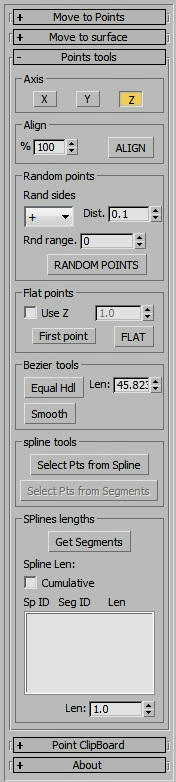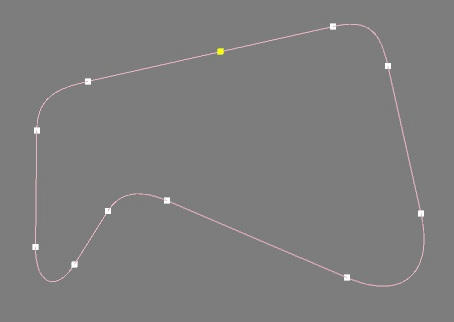## Build Track step by step

Pages: 1 | 2 | 3 | 4### Build reference splineTo create a track needs a line for center of track. This line will be called "Reference Spline".

The Reference Spline can have a number of points you want and wherever you want. Can be closed or open for example to make the access of pitbox need a open line.

For straight lines is better to have points at the beginning and end of the straight.

Can open Point Tools window in "Reference spline" section with PTtools button.

There are some functions to manipulate points in PointTools script.

Align Points:

Select the three points of the main straight to align.
Select the Axis X and Y if want to align the elevation turn on Z.
After press ALIGN the points will be moved to the line from the first point to the last. If points are bezier the bezier handles will align to.
The default 100% value means the percentage of the initial distance of each point. If the value is zero the point don't move.

Can use align to do a linear interpolation in elevation just need to turn on Z axis only.

Flat Points:

To flatten select points just select the points and press FLAT. The default Z value is taken from first point if press the button "First point" will change to "last point" and the last point ss the Z value. Can use a numeric value just need to check "Use Z" and write the value.

Bezier Tools:Bezier curves have tangent handles to generate curve. If we have two points to create the curve can use the button Equal Hdl (equal handles) do get the handles length equal and get a better curve.

The Snooth button are like max "reset tangents" but here only apply to selected axis. If select only Z axis is useful to smooth the bumps and irregularities.